Polygons and Angles

Polygons and Angles

You can use triangles to find the sum of the angle measures in other figures.

Step 1 Complete the table. Divide each figure into triangles by drawing as many diagonal lines as you can that begin at the point marked on the shape.

Step 2 Use your drawings to complete the table.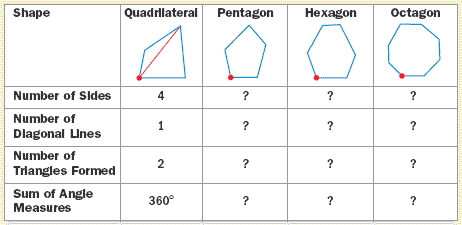Polygons
A polygon is a closed figure whose sides are line segments that intersect only at their endpoints. In a regular polygon, all the angles have the same measure, and all the sides have the same length.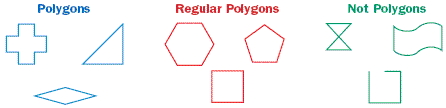Polygons can be identified by the number of their sides.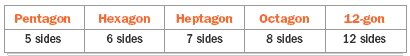Example

Identifying Figures

Is the figure a polygon, a regular polygon, or not a polygon? Explain.

a.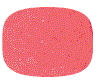Not a polygon. The figure does not have line segments as sides.

b.Regular polygon. Its angles have equal measures, and its sides have equal lengths.

Angles

In an earlier part of this lesson, you used triangles to find the sum of the angle measures in polygons. In a regular polygon, the measure of one angle is the sum of the angle measures divided by the number of sides.

Angle Measures in a Polygon with n sides (an n-gon):

Sum of angle measures in an n-gon: (n - 2)180Measure of one angle in a regular n-gon: ((n - 2)180)/ n

Example

Finding an Angle Measure
Find the measure of one angle in a regular octagon.

A regular octagon has 8 sides, so use n = 8.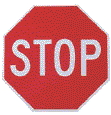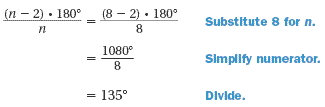The measure of one angle in a regular octagon is 135Practice

1. Complete the statement: A   ?   is a closed figure with sides that are line segments.

2. Are the following figures a polygon, a regular polygon, or not a polygon?
a.b.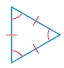c.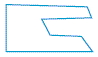3. Are the following swimming pool designs polygons?
a.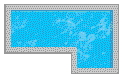b.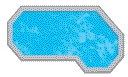c.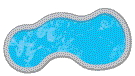4. Describe and correct the error in the solution.5. Find the measure of one angle in a regular 12-gon.
A. 30B. 60C. 120D. 1506. Find the sum of the angle measures in a nonagon (9-sided polygon).

7. Find the value of x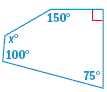8. Four angles in a pentagon measure 90, 85, 120, and 130What is the measure of the fifth angle?
A. 105B. 115C. 120D. 1659. The angles marked with letters are called exterior angles.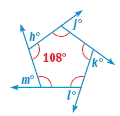a. Find the measures of the exterior angles of the polygon.

b. Find the sum of the exterior angle measures for the polygon.